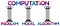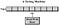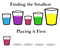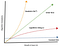# Quantum Computing for Newbies: Introduction to Computation

What is computation? Roughly stating, it means to solve a problem in an effective way after translating it into a suitable form according to a model.

I am pretty sure everyone has heard of quantum computing by now and has read about its superior capabilities than classical computing. Some got fascinated by it and started following this hot topic. Some went further to look up what this is. Some went even further to try to understand what really it is and then guess what happened: they got a headache.

Quantum computing can be very confusing for newbies who want a clear and brief understanding of it in simple and concise manner. There are several reasons for this confusion. Big one is, most don’t teach fundamental concepts required to grasp this or fail to deliver concepts in simple terms. Yes sure, you don’t need to be good at quantum mechanics to understand it, but you can’t get away with it without some concepts which are tied with quantum mechanics.

But even a bit of quantum mechanics can’t help you because you are missing the actual thing — computation. Any introduction to quantum computing will lead you nowhere if you don’t understand what computation is. Computation is the theoretical basis of computer science. The essence of computer science is not learning how to code or debug or think sequentially, but it is to understand what computation is. And let me tell you one thing — you can’t [fully] understand what computation is. Even computer scientists don’t understand it [fully] because there is no agreed definition of computation. Defining it is an ongoing process and kind of philosophical — more on this here.

So, here I would like to present a simple and brief introduction of computation. It may not be correct but it is closest I can get. One can immediately get the idea upon hearing computation that it has to do something with computing. But what is that we are computing? What is being computed and what for? A general understanding is, mathematical expressions (such as two numbers being added or subtracted or multiplied) are computed and they try to solve some kind of problem, which has been modeled in this form, in an effective manner.

Pause here, ponder over previous paragraph. You can notice we are heading to some profound concepts. Can you list them?

These concepts are: problem, model and effective manner (may be you remember a single word for this effective manner thing).

When we observe our world, we can see a lot of problems which demand our attention. To solve a problem, we try to first understand it. We create a representation of problem in our mind based on some assumptions. Then we try to come up with some steps to solve the problem which we perform one by one or even in parallel. The assumptions which we make constitute our model. The representation which we come up with using the model is the understandable form of problem. The solving steps become algorithm.Computation and its foundational concepts. Credits: Freepik/Milena B Design/Uihere

Let’s take an example here. Suppose you own a shop where you sell apples. At the beginning of a month, you bought one thousand apples for two thousand dollars. Now, you want to calculate the buying price of each apple so that you can make a good profitable selling price. This problem is quite simple and you can see mathematical model is best to solve this. So let’s translate it into a mathematical form. We can represent one thousand apples in decimal digit system as 1000. Next two thousand dollars as 2000. Also, we have to find buying price for each apple so we have to distribute 2000 on 1000. So you perform the division or give it to any child to perform it. He/she will give you answer of 2000/1000 as 2. Translating back this answer in our real world, we infer price of each apple is two dollars.

This is a very simple example and easy to understand. But I hope you noticed how we transformed this real world problem into a mathematical expression, which any one with knowledge of mathematical rules, can easily solve. So the computation in this example becomes computing the mathematical expression. There are other problems which we try to understand using different and more complex models such as exponential mathematical models for growth of population of a specie or Lotka-Voltera equations to model highly complex population dynamics of two interacting species, specifically predator and prey.

If you paid attention to the example, you would have noticed it mentioned something about giving the expression to the child to solve. Now, imagine instead of a child, you can design a machine which can compute mathematical expressions such as DMAS operations. So the only task you have at your hand is to translate your problems into mathematical expressions (using the mathematical model of course) and give it to the machine to compute. Then take the answer and infer its meaning in context of the real world. So, essentially what you are trying to do here is automate the process of computing the mathematical expression.

Here we turn to history. Before 1950s, computers were people who computed mathematical expressions and equations. Of course they were trained enough to even compute complex differential equations. But things were taking a turn at that moment. Invention of machines which could break encryption were at rise during World Wars. Concept of automatic computing was emerging which essentially meant giving a problem to a machine in suitable form and making it to compute for you.

To be able to translate the problem into machine suitable/readable form and come up with a procedure in which machine will work, new models were being presented. Among those models, prominent were Turing Machine, Finite State Automata and Lambda Calculus. The model which prevailed was Turing Machine because its assumptions were close to the workings of a digital computer.

So, let’s talk briefly about Turing Machine model of computation. It was proposed by Alan Turing in 1936. Simply stating, it is a machine which consists of a movable infinitely long tape on which symbols (specifically binary code) are written in neatly divided cells. It also has a fixed head which can read from the cell towards which it is pointing. Head can also manipulate the value it is reading based on a table of rules which contains instructions. Head can also write values onto any cell of the tape.A schematic diagram of Turing Machine. Credits: Jeff Stefan

You can clearly see that it is almost like a digital computer. A digital computer has a memory from which we can read and also write on it. A digital computer has a processor which can manipulate the read values based on rules which are also stored somewhere on memory. Only difference is that Turing Machine has infinitely long tape which gives it an infinite amount of memory but still calculations done for complexity of a computation on Turing Machine model are good estimate for digital computers also.

Apart from simple design, a Turing Machine is highly capable of solving many different problems. Almost all classical computing algorithms were based on assumptions similar to Turing Machine. For example take a simple sorting algorithm. Suppose you are given a list of 10 numbers which are distinct. Your task is to arrange them in ascending order. A human computer can do it with great ease. But can a Turing Machine do so?

Let’s translate this problem into a suitable form based on Turing Machine model. Let’s first write the 10 numbers on 10 consecutive cell of Turing Machine. Let’s call them cell 1, cell 2, cell 3 and so on till cell 10. We are going to write the arranged list of number in the cells immediately after the 10 consecutive cells. We can name them cell 11 till cell 20. We can write the instructions for the manipulations of cell values (the numbers of list) in the table of rules. These instructions (effective way to solve or algorithm) are:

1. At the beginning of process, place the head on cell 1. So head would be reading the value of cell 1.
2. Now move the head over to next cell, and compare the value in head (it is of cell 1 right now) with the value of cell 2. If cell 2’s value is smaller than head’s current value, then read the value of cell 2, otherwise don’t.
3. Move to next cell, and repeat step 2. Do this till you reach cell 10. At this point, you will have traversed the whole list and have the smallest value in the head.
4. Write the smallest value in cell 11.
5. Traverse the cells from 1 to 10 back and find the value which has been written in cell 11. Erase the value so that the cell becomes blank and its value doesn’t get written in new list more than once.
6. Repeat steps from 1 to 5 till you have completely written all values from cell 1 to cell 10 in cell 11 to cell 20. Now you will have an arranged list.

These instructions look tedious but they do the job well. Good thing about machines is that they are very fast. So using these instructions, a list containing 1000 values can be arranged in a very small time as compared to a human computer.Visualization of essence of algorithm. Credits: clipartmax

Above example demonstrates one example of how Turing Machine can be employed at solving a problem. You can solve a lot of other problems with it, if you can translate the problem in a suitable form for Turing Machine, keeping in mind model’s assumptions.

Now that we have discussed a little of bit of history also, we turn back to our discussion of computation. As we have tried to understand computation is an idea which is not explicitly defined in Computer Science instead keeps evolving as new discoveries are made. To understand it however you need to know the concepts of problem, model and algorithm which we have already discussed with examples.

Another concept in the realm of computation is of the computational complexity of a problem which can be simply understood as how complex a problem is to solve under a given model. Essentially, it depends on your effective way of or algorithm for solving the problem (which as we know itself depends on the model). The more steps/iterations/resources your algorithm will require to solve a problem, more complex it would be under that model. The notion of computational model is very important here. The whole hype around Quantum Computing has developed because this model of computation provides more effective ways to solve some problems as compared to Classical Computing. We will discuss difference between Classical and Quantum Computing at length in next article.

For now, to understand complexity more clearly we take example of complexity of a problem in Turing Machine model. Turing Machine requires two resources to work: time and space. Usually time is replaced by a more abstract and general concept of number of steps used by algorithm. The algorithm which we developed for arranging the list in ascending order requires only 20 spaces on the tape in total. We can say amount of space required by this algorithm is comparable to size of the list because assuming we have 25 elements in the list, then amount of space required would be 50. So there is a constant multiple or linear growth in case of space.

On the other hand, we require about 100 steps to arrange the list because each time we traverse the whole list to find the smallest value, we would be using about 10 steps and we traverse the list exactly 10 times. Now what if size of the list was 25. Then surely we would need about $25 \times 25 = 625$ steps. Hence, we notice a squared growth in number of steps to solve the problem as the size of problem grows. So the space complexity of this problem is linear while time complexity is quadratic essentially showing that we need more resources in time as compared to space.Comparison of growth w.r.t. problem size of various complexities. Credits: Jhantelle Belleza/Swift Algorithms and Data Structures by Wayne Bishop

At this point, we would wrap our discussion on computation. In this article we learned about computation which roughly means to solve a problem in an effective way after translating it into a suitable form according to a model. From next article, we will begin discussion of Classical Computing model and how Quantum Computing model differs from it.

Qubits Demystified is a channel by duo of Habib University students, Muhammad Ahmed and Raza Naqvi, to explain Quantum Computing in easy and concise way. Send feedback or write back to us at qubitsdemystified@gmail.com or hit us up on twitter at @QubitsD.

Channel explaining Quantum Computing in clear & concise language.